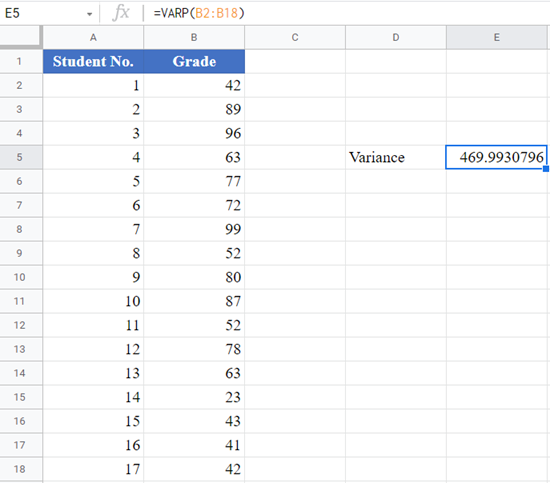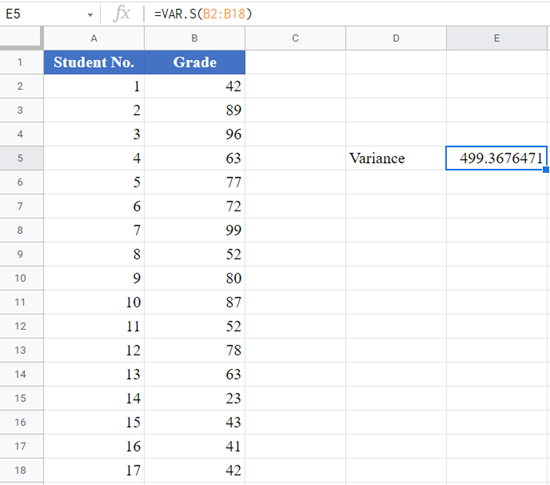# VAR.P vs VAR.S (Population vs Sample Variance) – Excel and Google Sheets

The tutorial will discuss the differences between Population and Sample Variance and demonstrate how to calculate each in Excel and Google Sheets.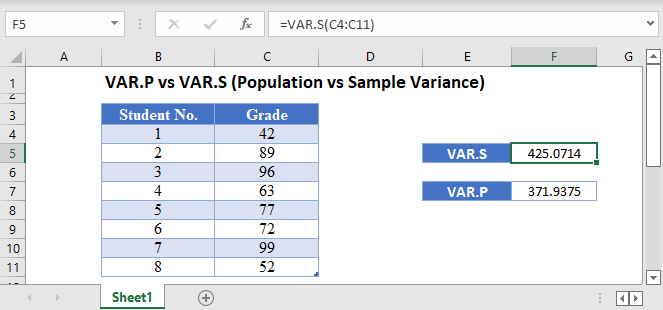Variance is a measure of the spread of a data distribution. It measures the distance of the data points from the mean of the dataset. The smaller the variance of a dataset, then the closer is the numbers in the dataset to each other.

There are two formulas used to calculate the variance of a data set:

• The VAR.P Function is used to calculate the variance of a dataset if the dataset is considered to be from the entire population.
• The VAR.S Function is used to calculate the variance of a dataset if the dataset is considered to be from a sample of a populate.

## VAR.P vs VAR.S – The Difference

The VAR.P Function is based on the formula: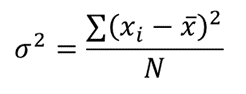Where σ2 is the population variance;

∑ is a Greek letter called sigma which represents ‘sum’;

xi represents each data point;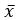is the mean (average) of the dataset; and

N is the population size (the number of data points in the population).

The VAR.S Function is based on the formula: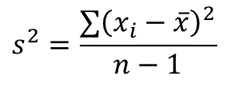Where s2is the sample variance;

∑ is a Greek letter called sigma which represents ‘sum’;

xi represents each data point;is the mean (average) of the dataset; and

n is the sample size (the number of data points in the sample).

Since the divisor in the population variance formula is N which is greater than the divisor for the sample variance formula, n-1, the population variance will always be smaller than the sample variance when both are calculated using the same dataset.

## When to Use VAR.P vs VAR.S Functions

Usually, we would like to calculate the variance of all the data points in the population of interest. However, we don’t have access to all the data points in the population. So, we select a subset of the population of interest which we refer to as the sample. With the sample, we then try to approximate the variance of the population using the sample.

Use the VAR.P Function when:

• Your dataset represents all the data points of the population of interest; or
• Your dataset represents the sample of a population, however, you do not wish to use your findings to generalize (estimate) the population.

Use the VAR.S Function when your dataset represents the sample of a population and you wish to use your findings to generalize (estimate) the population. This is the case most of the time.

## How to Use the VAR.P and VAR.S Functions in Excel

### VAR.P Function

Background: Ms. Fatima teaches Statistics 101 at a local college. The table below shows the percentage grades of the 2021 Statistics 101 Fall semester students. Calculate the variance of the percentage grades of Ms. Fatima’s 2021 Statistics 101 Fall semester students.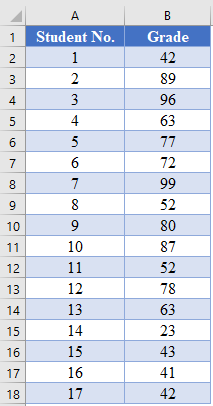Here, we are interested in only Ms. Fatima’s 2021 Statistics 101 Fall semester students and the given dataset contains all the grades of Ms. Fatima’s 2021 Statistics 101 Fall semester students. So, the given dataset is treated as the population of interest. Thus, we use the VAR.P Function to calculate the variance as shown in the picture below.

``=VAR.P(B2:B18)``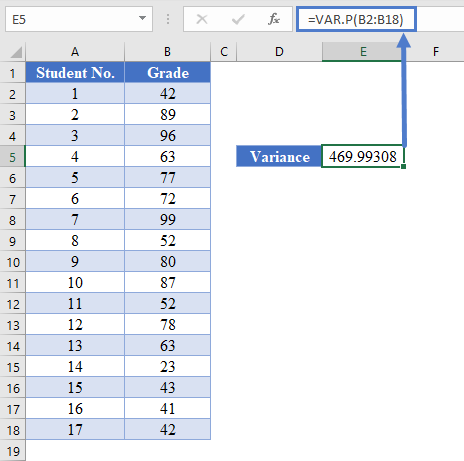### VAR.S Function

Background: Ms. Fatima teaches Statistics 101 at a local college. The table below shows the percentage grades of the 2021 Statistics 101 Fall semester students. Use the data to estimate the variance of the percent grades of Ms. Fatima’s Statistics 101 students.Here, we are interested in all of Ms. Fatima’s Statistics 101 students. However, we only have information about Ms. Fatima’s Statistics 101 2021 Fall semester students which is a subset of all Ms. Fatima’s Statistics 101 students. So, the given dataset is treated as a sample from the population of interest. Thus, we use the VAR.S Function to calculate the variance as shown in the picture below.

``=VAR.S(B2:B18)``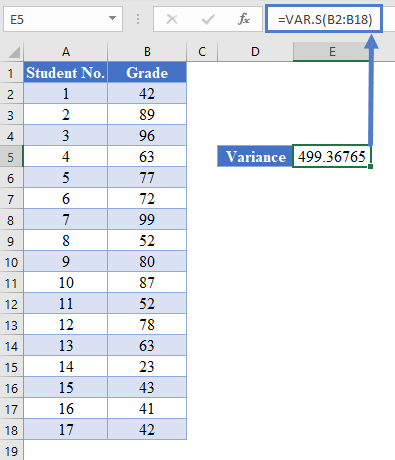## VARP vs VARS in Google Sheets

The VAR.P and VAR.S Functions are used in Google Sheets in the exact same way as they are used in Excel, except they are called VARP and VARS.